## Example Questions

← Previous 1

### Example Question #1 : How To Multiply Square Roots

Simplify: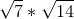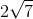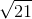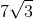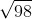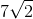Explanation:

When multiplying square roots, you are allowed to multiply the numbers inside the square root. Then simplify if necessary.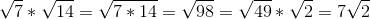### Example Question #2 : How To Multiply Square Roots

Simplify: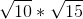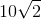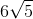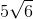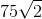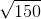Explanation:

When multiplying square roots, you are allowed to multiply the numbers inside the square root. Then simplify if necessary.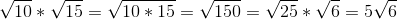### Example Question #1 : How To Multiply Square Roots

Simplify: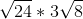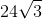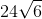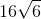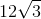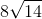Explanation:

When multiplying square roots, you are allowed to multiply the numbers inside the square root. Then simplify if necessary.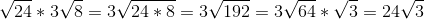### Example Question #4 : How To Multiply Square Roots

Simplify: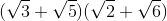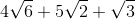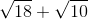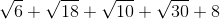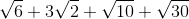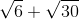Explanation:

To simplify the problem, just distribute the radical to each term in the parentheses.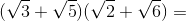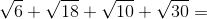### Example Question #5 : How To Multiply Square Roots

Evaluate and simplify: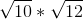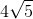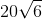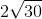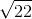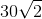Explanation:

To multiply square roots, we multiply the numbers inside the radical and we can simplify them if possible.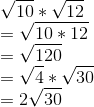### Example Question #6 : How To Multiply Square Roots

Simplify and evaluate: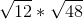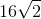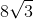Explanation:

To multiply square roots, we multiply the numbers inside the radical and we can simplify them if possible.

In this case, let's simplify each individual radical and multiply them.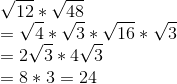### Example Question #7 : How To Multiply Square Roots

Simplify: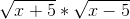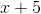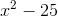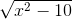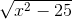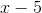Explanation:

To multiply square roots, we multiply the numbers inside the radical and we can simplify them if possible.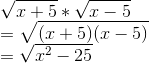### Example Question #8 : How To Multiply Square Roots

Simplify: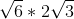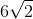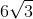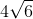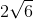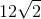Explanation:

To multiply square roots, we multiply the numbers inside the radical.

Any numbers outside the radical are also multiplied. We can simplify them if possible.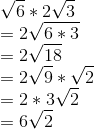### Example Question #9 : How To Multiply Square Roots

Simplify: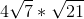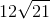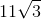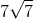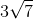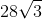Explanation:

To multiply square roots, we multiply the numbers inside the radical.

Any numbers outside the radical are also multiplied.

We can simplify them if possible.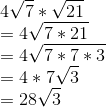### Example Question #10 : How To Multiply Square Roots

Simplify: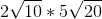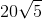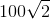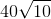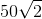Explanation:

To multiply square roots, we multiply the numbers inside the radical.

Any numbers outside the radical are also multiplied.

We can simplify them if possible.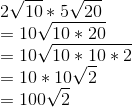← Previous 1